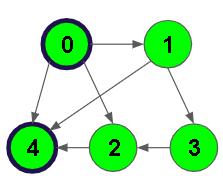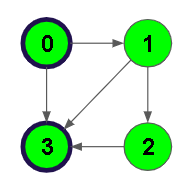XDAYS

:

HOUR

:

MINS

:

SEC

Copied to Clipboard
Possible paths between 2 vertices
Medium Accuracy: 52.95% Submissions: 12547 Points: 4

Given a Directed Graph having V nodes numbered from 1 to V, and E directed edges. Given two nodes, source and destination, count the number of ways or paths between these two vertices in the directed graph. These paths should not contain any cycle.
Note: Graph doesn't contain multiple edges, self-loop, and cycles.

Example 1:

Input:
V = 5, E = 7
Edges of Graph =
{0, 1}
{0, 2}
{0, 4}
{1, 3}
{1, 4}
{2, 4}
{3, 2}
source = 0
destination = 4

Output: 4
Explanation:There are 4 paths from 0 to 4.
0 -> 4
0 -> 1 -> 4
0 -> 2 -> 4
0 -> 1 -> 3 -> 2 -> 4


Example 2:

Input:
V = 4, E = 5
Edges of Graph =
{0, 1}
{0, 3}
{1, 2}
{1, 3}
{2, 3}
source = 0
destination = 3
Output: 3
Explanation:There are 3 paths from 0 to 3.
0 -> 3
0 -> 1 -> 3
0 -> 1 -> 2 -> 3


You don't need to read, input, or print anything. Your task is to complete the function countPaths(), which takes the integer V denoting the number of vertices, adjacency list adj, integer source, and destination as input parameters and returns the number of paths in the graph from the source vertex to the destination vertex.

Expected Time Complexity: O(V!)
Expected Auxiliary Space: O(V)

Constraints:
1 ≤ V, E ≤ 100
1 ≤ source, destination ≤ V

We are replacing the old Disqus forum with the new Discussions section given below.

### Editorial

We strongly recommend solving this problem on your own before viewing its editorial. Do you still want to view the editorial?

#### My Submissions: